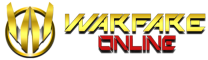# Treasure Box Spawn Coordinates

Treasure boxes can be of great use for Warfarians as they contain Pearls, Ancient Pearls, Jewelry Ang Pau’s, Golden Jewelry Ang Pau’s, 5M EXP balls and Tears of Sparda (TOS).

The ruthless lands inhabiting powerful creatures of Sainna Island, Misery Island and Caernarvon are where these treasure boxes are found. Discover the Treasure boxes at these coordinates of the respective maps given below. However, keep in mind that the spawn time of the treasure boxes are random.

`Note: Up to 24 treasure boxes are spawned within an hour in Caernarvon, Sainna Island and Misery Island respectively. However, not all 24 boxes will spawn at once and the spawn times will always differ. `

(1462,1249)
(2743,1189)
(2030,3475)
(1640,3111)
(2435,3154)
(2030,2718)
(1050,2091)
(3473,2546)
(678,2900)
(3427,3288)
(1118,3030)
(2871,3260)
(1670,1406)
(2710,1690)
(2030,2100)
(1450,2200)
(2540,2545)
(2240,1336)
(1065,903)
(3070,1356)
(2211,720)
(864,1311)
(3164,1760)

(1573,2325)
(1036,884)
(1445,1774)
(1764,1656)
(2192,1287)
(1430,820)
(1032,1273)
(1248,923)
(1223,2222)
(850,1263)
(1799,2192)
(1632,1927)
(1263,692)
(525,968)
(1784,1125)
(1159,1218)
(1258,1882)
(1071,2148)
(2094,1873)
(2040,1528)
(933,707)
(791,1474)
(1907,1076)
(1671,1174)
(1405,2089)
(1046,1961)
(2364,1892)
(1479,1435)
(997,1105)
(1159,1632)
(2050,1233)
(1479,1100)

(1251,1038)
(1927,1708)
(1787,782)
(788,1732)
(2444,1616)
(2895,898)
(2085,2718)
(2560,2055)
(1421,928)
(1756,1598)
(2615,2846)
(1257,2432)
(2042,1921)
(2718,1373)
(1823,2091)
(2304,2365)
(934,940)
(952,1561)
(971,2402)
(825,2213)
(2396,2012)
(2956,1269)
(1854,2584)
(2755,2292)
(2116,1726)
(1933,611)
(1208,2006)
(1641,1939)
(3078,1166)
(1969,2889)
(1835,2359)
(2493,2323)

3 Likes